NEET  >  Previous year questions (2014-22): Laws of Motion

# Previous year questions (2014-22): Laws of Motion - Notes | Study Physics Class 11 - NEET

 1 Crore+ students have signed up on EduRev. Have you?

Q.1. An electric lift with a maximum load of 2000 kg (lift + passengers) is moving up with a constant speed of 1.5 ms–1. The frictional force opposing the motion is 3000 N. The minimum power delivered by the motor to the lift in watts is : (g = 10 m s–2 )           

(1) 20000
(2) 34500
(3) 23500
(4) 23000

Ans: 2
Fup = 2000g + 3000
= 23000 N
Minimum power Pmin =  F v
= 23000 * 3/2
= 34500 W

Q.2. A ball of mass 0.15 kg is dropped from a height 10 m, strikes the ground and rebounds to the same height. The magnitude of impulse imparted to the ball is (g = 10 m/s2) nearly :     
A: 2.1 kg m/s
B: 1.4 kg m/s
C: 0 kg m/s
D: 4.2 kg m/s
Ans:
D
Solution:
Velocity just before striking the groundIf it reaches the same height,speed remains same after collision only the direction changes.Q.3. Two bodies of mass 4 kg and 6 kg are tied to the ends of a massless string. The string passes over a pulley which is frictionless (see figure). The acceleration of the system in terms of acceleration due to gravity (g) is :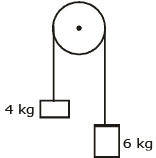A: g/5B: g/10
C: g
D: g/2

Ans: A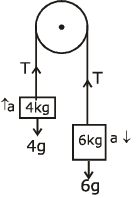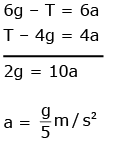Q 4.  A block of mass 10 kg is in contact against the inner wall of a hollow cylindrical drum of radius 1 m. The coefficient of friction between the block and the inner wall of the cylinder is 0.1. The minimum angular velocity needed for the cylinder to keep the block stationary when the cylinder is vertical and rotating about its axis, will be : (g 10 m/s2)     
Ans:
C
Solution: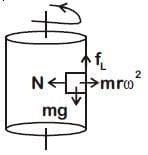For equilibrium of the block limiting friction
fL≥ mg
⇒ μN ≥ mg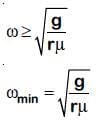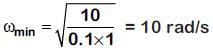Q 5. A mass m is attached to a thin wire and whirled in a vertical circle. The wire is most likely to break when:    
A: The mass is at the highest point
B: The wire is horizontal
C: The mass is at the lowest point
D: Inclined at an angle of 60° from vertical
Ans:
C:
Solution: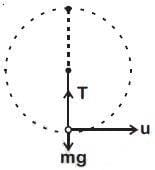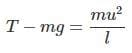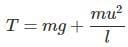The tension is maximum at the lowest position of mass, so the chance of breaking is
maximum.

Q 6. When an object is shot from the bottom of a long smooth inclined plane kept at an angle 60° with horizontal, it can travel a distance x1 along the plane. But when the inclination is decreased to 30° and the same object is shot with the same velocity, it can travel x2 distance.
Then x1 : x2 will be:    
A: 1: √2
B: √2: 1
C: 1: √3
D: 1: 2√3
Ans:
C
Solution: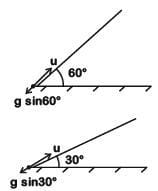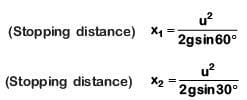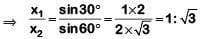Q 7.Which one of the following statements is incorrect ?
A: Rolling friction is smaller than sliding friction
B: Limiting value of static friction is directly proportional to normal reactions
C: Frictional force opposes the relative motion
D: Coefficient of sliding friction has dimensions of length    
Ans:
D
Solution: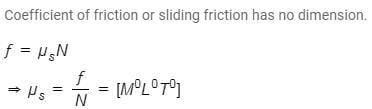Q 8. A block of mass m is placed on a smooth inclined wedge ABC of inclination θ as shown in the figure. The wedge is given an acceleration 'a' towards the right. The relation between a and θ for the block to remain stationary on the wedge is :-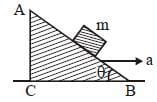A: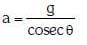B: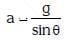C: a = g cos θ
D: a = g tan θ
Ans:
D
Solution: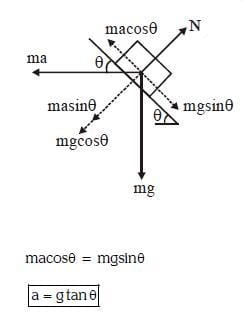Q 9. Two blocks A and B of masses 3 m and m respectively are connected by a massless and inextensible string. The whole system is suspended by a massless spring as shown in figure. The magnitudes of acceleration of A and B immediately after the string is cut, are respectively :-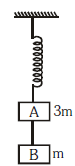A: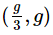B: g, g
C: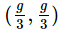D: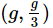Ans:
A
Solution: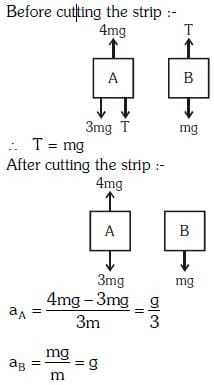Q 10. What is the minimum velocity with which a body of mass m must enter a vertcal loop of radius R so that it can complete the loop ? 
A: √5gR
B: √gR
C: √2gR
D: √3gR
Ans:
A
Solution:
The question is illustrated in the figure below,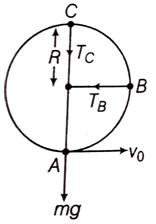Let, the tension at point A be TA.
Using Newton's second law, we have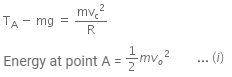Energy at point C is,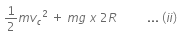At point C, using Newton's second law,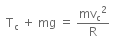In order to complete a loop, T≥ 0
so,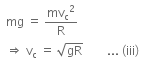From equation (i) and (ii)
Using the principle of conservation of energy,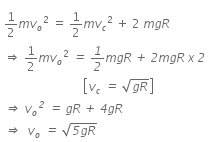Q 11. A particle of mass 10 g moves along a circle of radius 6.4 cm with a constant tangential acceleration. What is the magnitude of this acceleration if the kinetic energy of the particle becomes equal to 8 x 10-4J by the end of the second revolution after the beginning of the motion ?    
A: 0.2 m/s2

B: 0.1 m/s2
C: 0.15 m/s2
D: 0.18 m/s2
Ans: B
Solution:

Given, mass of particle. m = 0.01 kg
Radius of circle along which particle is moving , r = 6.4 cm
Kinetic energy of particle, K.E. = 8 x 10-4 J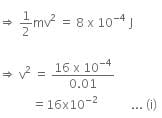Given that, KE of particle is equal to 8 x 10-4 J by the end of second revolution after the beginning of the motion of particle.
It means, initial velocity (u) is 0 m/s at this moment.
Now, using the Newton's 3rd equation of motion,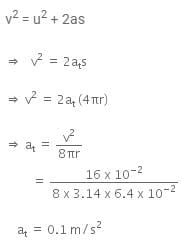Q 12. A car is negotiating a curved road of radius R. The road is banked at an angle .. The coefficient of friction between the tyres of the care and the road is 1s. The maximum safe velocity on this road is:    
A: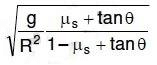B: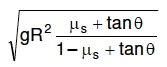C: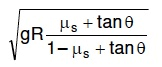D: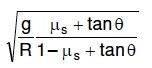Ans:
C
Solution: A car is negotiating a curved road of radius R. The road is banked at angle straight theta and the coefficient of friction between the tyres of car and the road is straight mu subscript straight s.
The given situation is illustrated as: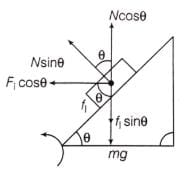In the case of vertical equilibrium,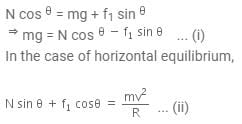Dividing Eqns. (i) and (ii), we get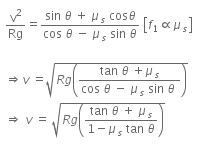Q 13. Three blocks A, B and C, of masses 4 kg, 2 kg and 1 kg respectively, are in contact on a frictionless surface, as shown. If a force of 14 N is applied on the 4 kg block, then the contact force between A and B is :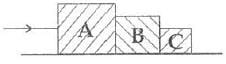A: 18 N
B: 2 N
C: 6 N
D: 8 N
Ans:
C
Solution: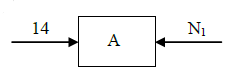a =14/7 = 2m/s2
∴ 14 - N1 = 4 x 2
N1 = 6N

Q 14. A rod of weight W is supported by two parallel knife edges A and B and is in equilibrium in a horizontal position. The knives are at a distance d from each other. The centre of mass of the rod is at distance x from A. The normal reaction on A is :    
A: W(D-x)/d
B: Wx/d
C: Wd/x
D: W(d-x)/x
Ans:
A
Solution: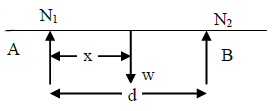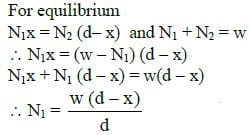Q 15. A particle of mass m is driven by a machine that delivers a constant power k watts. If the particle starts from rest the force on the particle at time t is :    
A: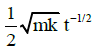B: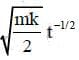C: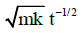D: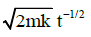Ans:
C
Solution: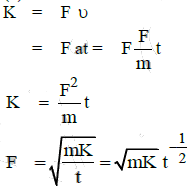Q 16. A block A of mass m1 rests on a horizontal table. A lights string connected to it passes over a frictionless pulley at the edge of table and from its other end another block B of mass m2 is suspended. The coefficient of kinetic friction between the block and the table is μk. When the block A is sliding on the table, the tension in the string is:   
A: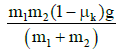B: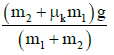C: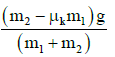D: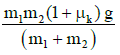Ans:
D
Solution: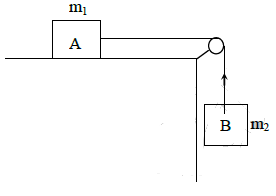See figure alongside
Let T be the tension in the string.
Let a be the acceleration of the combination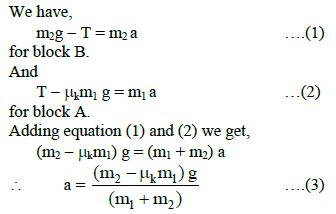From Equation (2) and (3) we get,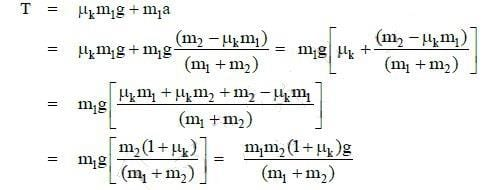Q 17. A balloon with mass ‘m’ is descending down with an acceleration ‘a’(where a<g). How much mass should be removed from it so that it starts moving up with an acceleration ‘a’ ?    
A: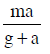B: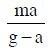C: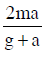D: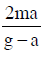Ans: C
Solution:
Let the up thrust on balloon be U.
mq - U = ma     ...(i)
If Δm is removed
U = (m - Δm)g = (m - Δm)a    ..(ii)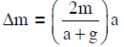Q 18. A system consists of three masses m1, m2 and m3 connected by a string passing over a pulley P. The mass m1 hangs freely and m2 and m3 are on a rough horizontal table (the coefficient of friction = μ). The pulley is frictionless and of negligible mass. The downward acceleration of mass m1 is :
(Assume m1 = m2 = m3 = m)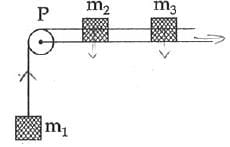A: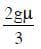B: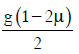C: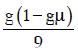D: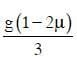Ans:
D
Solution: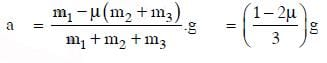Q 19. The force F acting on a particle of mass m is indicated by the force-time graph as shown below. The climate change in momentum of the particle over the time interval from zero to 8s is,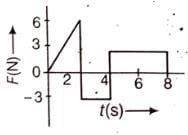A: 12 Ns
B: 6 Ns
C: 24 Ns
D: 20 Ns
Ans:
A
Solution: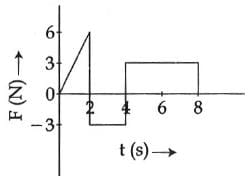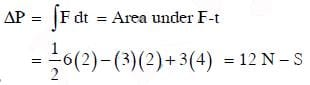The document Previous year questions (2014-22): Laws of Motion - Notes | Study Physics Class 11 - NEET is a part of the NEET Course Physics Class 11.
All you need of NEET at this link: NEET

## Physics Class 11

127 videos|464 docs|210 tests
 Use Code STAYHOME200 and get INR 200 additional OFF

## Physics Class 11

127 videos|464 docs|210 tests

### How to Prepare for NEET

Read our guide to prepare for NEET which is created by Toppers & the best Teachers

Track your progress, build streaks, highlight & save important lessons and more!

,

,

,

,

,

,

,

,

,

,

,

,

,

,

,

,

,

,

,

,

,

;